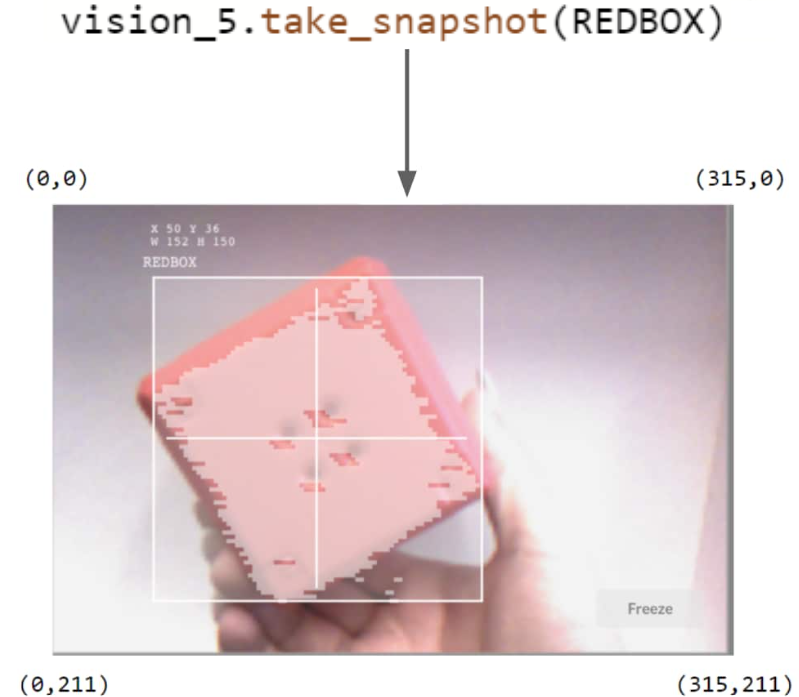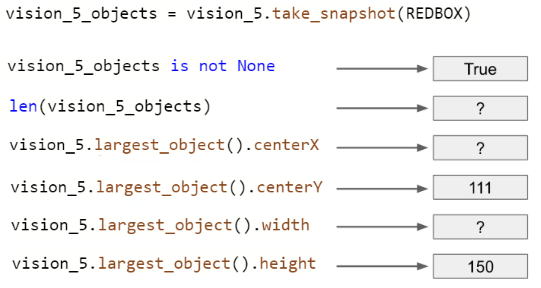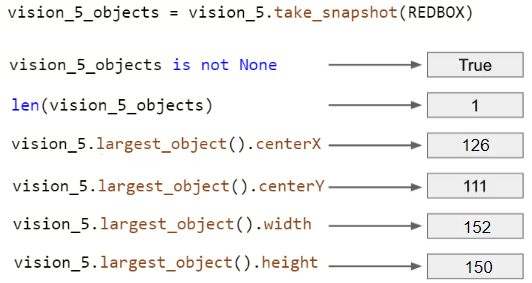STEMLabs V5
Vision Sensor Teacher

Teacher PortalTeacher Toolbox - The Purpose of this Activity

This activity has students apply what they learned on the previous page in order to complete a data set from an example snapshot. They will fill in missing values, calculate center X and Y values, and interpret what the data can tell us about the object's position relative to the robot's center point. This will prepare them for success on the Vision Data Challenge that follows.Here are the provided data from the snapshot:

• X = 50

• Y = 36

• W = 152

• H = 1501. Is the REDBOX to the left or to the right of the robot's center point?

2. Is the REDBOX higher or lower than the robot's center point?Teacher Tips

Draw the students' attention to the fact that the detection frame does not fully cover the REDBOX. That is okay. It still recognized the REDBOX. Tuning the Vision Sensor is unlikely to perfect the detection frame and that is ok. The Vision Sensor does recognize the majority of the REDBOX.Teacher Toolbox - Answers

Students' answers can be discussed as a class and/or you can review their engineering notebooks to ensure that they completed the activity.

There is only one object (object count = 1) in the snapshot and the width is provided by the program (object width = 152). The center X value is 152/2 + 50 = 126.1. The REDBOX is to the left of the robot's center point (a bit left of center). The REDBOX is 31.5 pixels (center 157.5 - 126) left of the robot's center point.

2. The REDBOX is lower than the robot's center point (slightly lower than center). The REDBOX is 5.5 pixels (111 - center 105.5) below the robot's center point.